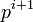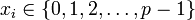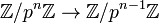View other such prime-parametrized groups

## Definition

Let$p$ be a (fixed here) prime number. This is a group determined uniquely up to isomorphism based on$p$ and is sometimes denoted$\mathbb{Z}_{(p)}$ (though that notation is also used for other things, and we can best infer meaning from context).

### As an inverse limit

The additive group of p-adic integers is a profinite group defined as the inverse limit of the inverse system:$0 \leftarrow \mathbb{Z}/p\mathbb{Z} \leftarrow \mathbb{Z}/p^2\mathbb{Z} \leftarrow \dots \leftarrow \mathbb{Z}/p^n\mathbb{Z} \leftarrow \dots$

where each of the maps:$\mathbb{Z}/p^{n-1}\mathbb{Z} \leftarrow \mathbb{Z}/p^n\mathbb{Z}$

reduces an integer mod$p^n$ to its value mod$p^{n-1}$.

Note that this definition also endows the group with a topology as a profinite group. In this topology, two elements are close if they agree mod$p^n$ for large$n$.

### As sequences with cumulative information$(a_0, a_1, a_2, \dots, a_n, \dots)$

where$a_i$ is an integer mod$p^{i+1}$, the addition is coordinate-wise (with each coordinate addition in the integers mod$p^{i+1}$), and for$i < j$, reducing$a_i$ mod$p^{j+1}$ yields$a_j$.

### As sequences with carries

The additive group of p-adic integers is the set of formal sums:$\sum_{i=0}^\infty x_ip^i$

where$x_i \in \{ 0,1,2,\dots,p-1 \}$, and the addition is done with carries, i.e., to add two sequences$\sum_{i=0}^\infty x_ip^i$ and$\sum_{i=0}^\infty y_ip^i$, we add coordinate-wise and if any of the sums is$p$ or more, we take a carry of 1 to the next sum.

### As the additive group of the ring of Witt vectors

This group is the additive group of the ring of Witt vectors over the prime field$\mathbb{F}_p$.

## Arithmetic functions

Function Value Explanation
order of a profinite group$p^\infty$ for the relevant prime$p$. This is a supernatural number as is the convention for orders of profinite groups.

## Powering

The additive group of$p$-adic integers is powered over the set of all primes other than$p$.

## Group properties

### Abstract group properties

Property Satisfied? Explanation
abelian group Yes
aperiodic group Yes No nonzero element has finite order.

### Topological group properties

Here, the topology is from the profinite group structure.

Property Satisfied? Explanation
profinite group Yes By definition
pro-p-group Yes By definition
compact group Yes profinite groups are compact
T0 topological group Yes profinite groups are Hausdorff, hence$T_0$
totally disconnected group Yes profinite groups are totally disconnected
topologically cyclic group Yes$\mathbb{Z}$, the subgroup generated by the element that projects to 1 mod$p^n$ for all$n$, is a dense subgroup. In fact, this group can be thought of as a compactification of$\mathbb{Z}$.
topologically finitely generated group Yes Follows from being topologically cyclic.
connected topological group No It is nontrivial and totally disconnected.

## Related notions

### Combining all primes

The profinite completion of the integers, denoted$\hat {\mathbb{Z}}$ is a group obtained by taking the profinite completion of the group of integers, which is a residually finite group. Intuitively, this group is obtained by "completing" the group of integers simultaneously at all primes. It turns out that the profinite completion of the integers can be viewed as the external direct product, over all primes$p$, of the additive group of$p$-adic integers.

### Dual concept

The additive group of p-adic integers can, in a vague sense, be considered to be constructed using a method dual to the method used to the quasicyclic group. While the$p$-adics are constructed as an inverse limit for surjective maps$\mathbb{Z}/p^n\mathbb{Z} \to \mathbb{Z}/p^{n-1}\mathbb{Z}$, the quasicyclic group is constructed as a direct limit for injective maps$\mathbb{Z}/p^{n-1}\mathbb{Z} \to \mathbb{Z}/p^n\mathbb{Z}$.